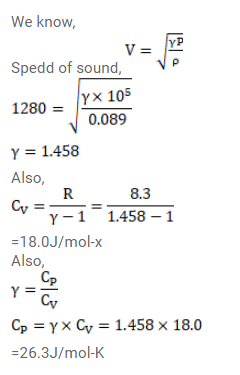# The speed of sound in hydrogen at 0°C isQuestion:

The speed of sound in hydrogen at $0^{\circ} \mathrm{C}$ is $1280 \mathrm{~m} / \mathrm{s}$. The density of hydrogen at STP is $0.089 \mathrm{~kg} / \mathrm{m}^{3}$. Calculate the molar heat capacities $C_{p}$ and $C_{v}$ of hydrogen.

Solution: